### This is Minnesota

This is Minnesota.  When I first arrived here in late June, the locals were complaining about the cold spring and the day's high temperature of 59 degrees.  Complaining about the cold, it seems, is yesterday's news.

Today we're enduring the heat.  Having lived in The South for the past 12 years, I thought I knew what heat was.  Apparently, I did not.  Apparently, I had to come back to Minnesota to figure that out.

Today we set an all time Minnesota high dewpoint of 83 degrees.  That is the temperature at which condensation on your windows and glasses will occur.  It's also as low as the thermometer is going to get tonight.

Combine the forecasted high of 100 degrees fahrenheit with a dew point in the 80's and you will come up with a heat index of well over 120 degrees.  If you don't believe me, you can figure it out yourself: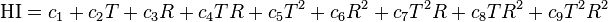$\mathrm{HI} = c_1 + c_2 T + c_3 R + c_4 T R + c_5 T^2 + c_6 R^2 + c_7 T^2R + c_8 T R^2 + c_9 T^2 R^2\ \,$
where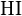$\mathrm{HI}\,\!$ = heat index (in degrees Fahrenheit)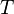$T\,\!$ = ambient dry-bulb temperature (in degrees Fahrenheit)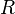$R\,\!$ = relative humidity (in percent)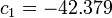$c_1 = -42.379 \,\!$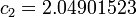$c_2 = 2.04901523\,\!$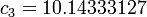$c_3 = 10.14333127\,\!$$c_4 = -0.22475541\,\!$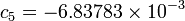$c_5 = -6.83783 \times 10^{-3}\,\!$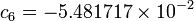$c_6 = -5.481717 \times 10^{-2}\,\!$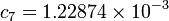$c_7 = 1.22874\times 10^{-3}\,\!$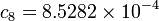$c_8 = 8.5282 \times 10^{-4}\,\!$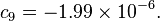$c_9 = -1.99 \times 10^{-6}.\,\!$]

I'm not kidding.  This is the real formula.
Who knew all those weathermen had to know so much calculus?

In simple terms, it means that walking outside will rival walking through the rain forest.

Only instead of Tarzan and monkeys and vines, there are cornfields.
Cornfields that are blanketed in a hot, sweaty haze.

What they say up here is this:  If you don't like the weather, just wait a few days.
Or wait six months when it will feel 160 degrees colder!